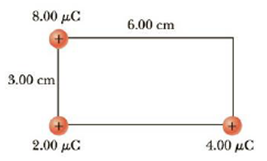Chapter 16, Problem 13P

Chapter
Section
Textbook Problem

(a) Find the electric potential, taking zero at infinity, at the upper right corner (the corner without a charge) of the rectangle in Figure P16.13. (b) Repeat if the 2.00-μC charge is replaced with a charge of −2.00 μC.Figure P16.13 Problems 13 and 14.

(a)

To determine
The electric potential.

Explanation

Formula to calculate the potential is,

V=keq1r1+keq2r2+keq3r12+r22

• ke is the Coulomb constant.
• q1 , q2 and q3 are the charges.
• r1 , r2 are the distances of charges from the point.

Substitute 8.00μC for q1 , 4.00μC for q2 , 2.00μC for q3 , 6.00 cm for r1 ,2.00 cm for r2 and 8.99×109 Nm2C2 for ke

(b)

To determine
The electric potential.

Still sussing out bartleby?

Check out a sample textbook solution.

See a sample solution

The Solution to Your Study Problems

Bartleby provides explanations to thousands of textbook problems written by our experts, many with advanced degrees!

Get Started

Describe diffusion in terms or entropy.

Biology: The Unity and Diversity of Life (MindTap Course List)

What are sister chromatids?

Human Heredity: Principles and Issues (MindTap Course List)

What is the monomer of a nucleic acid macromolecule?

Biology: The Dynamic Science (MindTap Course List)